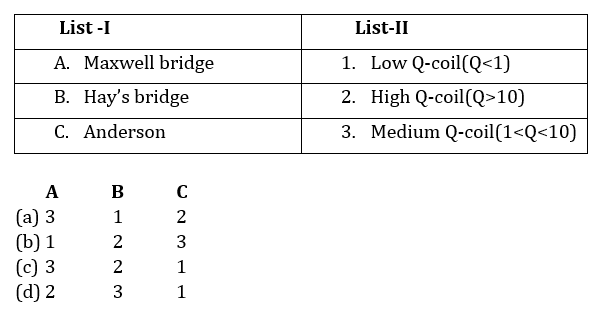# DFCCIL’21 EE: Daily Practices Quiz 19-July-2021

Each question carries 1 mark.
Negative marking: 1/4 mark
Total Questions: 06
Time: 08 min.

Q1. The short circuit test in a transformer is used to determine……
(a) Eddy current
(b) Hysteresis loss
(c) Copper loss at any load
(d) Iron loss at any load

Q2. Two coils with self-inductances 1 H and 2 H having a mutual inductance of 1 H between them carry currents of 2 A and √2 A respectively. The energy stored in the field, in joules, is
(a) 2(1+√2)
(b) 3(1+√2)
(c) 2(2+√2)
(c) 3(2+√2)

Q3. Ferranti effect happens in a transmission line when line is
(d) none of the above

Q4. What is the ratio of starting torque and maximum torque of a 3-phase, 50 Hz, 4 pole induction motor for maximum torque at 1200 rpm?
(a) 0.5
(b) 0.6
(c) 0.421
(d) 0.384

Q5. If the gain of the open-loop system is doubled, the gain margin
(a) Becomes half
(b) Gets doubled
(c) Becomes one-fourth
(d) Is not affected

Q6. Match the followingSOLUTIONS
S1. Ans.(c)
Sol. SC test of transformer:
Used to determine copper loss or ohmic loss
LV side short circuited
Instruments kept on HV side
Also determines equivalent resistance and leakage reactance

S2. Ans.(c)
Sol. ACQ: L_1=1 H,L_2=2 H & M=1 H
& i_1=2 A,i_2=√2 A
Energy stored=[1/2 L_1 i_1^2+1/2 L_2 i_2^2+Mi_1 i_2 ]J
∴E=[1/2×1×4+1/2×2×2+1×2×√2]=4+2√2=2(2+√2) joules

S3. Ans.(b)
Sol.
Ferranti effect occurs for medium and long transmission line.
Due to Ferranti effect, receiving end voltage is more than sending end voltage.ie.V_R>V_S

S4. Ans.(d)
Sol. N_s=120f/P=(120×50)/4=1500 rpm
∴s=(N_s-N_r)/N_s =(1500-1200)/1500=0.2
∴T_st/T_m =2/(s+1/s)=2/(0.2+1/0.2)=2/5.2=0.384

S5. Ans.(a)
Sol. Gain margin is calculated at phase cross over frequency. At phase cross-over frequency phase of G(jω) is -180⁰.
GM=1/X
If X becomes doubled then the GM becomes half.

S6. Ans.(c)
Sol. Bridges used for measurement of inductance:
Anderson bridge (Q<1).
Maxwell bridge (1<Q<10).
Hay bridge (Q>10).

×Join India's largest learning destination

What You Will get ?

•Job Alerts
•Daily Quizzes
•Subject-Wise Quizzes
•Current Affairs
•Previous year question papers
•Doubt Solving session

ORJoin India's largest learning destination

What You Will get ?

•Job Alerts
•Daily Quizzes
•Subject-Wise Quizzes
•Current Affairs
•Previous year question papers
•Doubt Solving session

ORJoin India's largest learning destination

What You Will get ?

•Job Alerts
•Daily Quizzes
•Subject-Wise Quizzes
•Current Affairs
•Previous year question papers
•Doubt Solving session

Enter the email address associated with your account, and we'll email you an OTP to verify it's you.Join India's largest learning destination

What You Will get ?

•Job Alerts
•Daily Quizzes
•Subject-Wise Quizzes
•Current Affairs
•Previous year question papers
•Doubt Solving session

Enter OTP

Please enter the OTP sent to
/6

Did not recive OTP?

Resend in 60sJoin India's largest learning destination

What You Will get ?

•Job Alerts
•Daily Quizzes
•Subject-Wise Quizzes
•Current Affairs
•Previous year question papers
•Doubt Solving sessionJoin India's largest learning destination

What You Will get ?

•Job Alerts
•Daily Quizzes
•Subject-Wise Quizzes
•Current Affairs
•Previous year question papers
•Doubt Solving session

Almost there

+91Join India's largest learning destination

What You Will get ?

•Job Alerts
•Daily Quizzes
•Subject-Wise Quizzes
•Current Affairs
•Previous year question papers
•Doubt Solving session

Enter OTP

Please enter the OTP sent to Edit Number

Did not recive OTP?

Resend 60

By skipping this step you will not recieve any free content avalaible on adda247, also you will miss onto notification and job alerts

Are you sure you want to skip this step?

By skipping this step you will not recieve any free content avalaible on adda247, also you will miss onto notification and job alerts

Are you sure you want to skip this step?